您可以捐助，支持我们的公益事业。 1元 10元 50元 认证码：必填求知 文章 文库 Lib 视频 iProcess 课程 认证 咨询 工具 讲座 Modeler Code要资料订阅捐助
Python数据挖掘与机器学习，快速掌握聚类算法和关联分析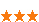1292 次浏览     评价： 好 中 差
2018-5-14

 编辑推荐: 来源 阿里云云栖社区，文中将为大家介绍聚类算法和关联分析问题。分类算法与聚类到底有何区别？聚类方法应在怎样的场景下使用？如何使用关联分析算法解决个性化推荐问题？本文就为大家揭晓答案。

K-Means算法是一种经典的聚类算法，也称作K均值聚类算法，先为大家介绍K-Means算法的原理。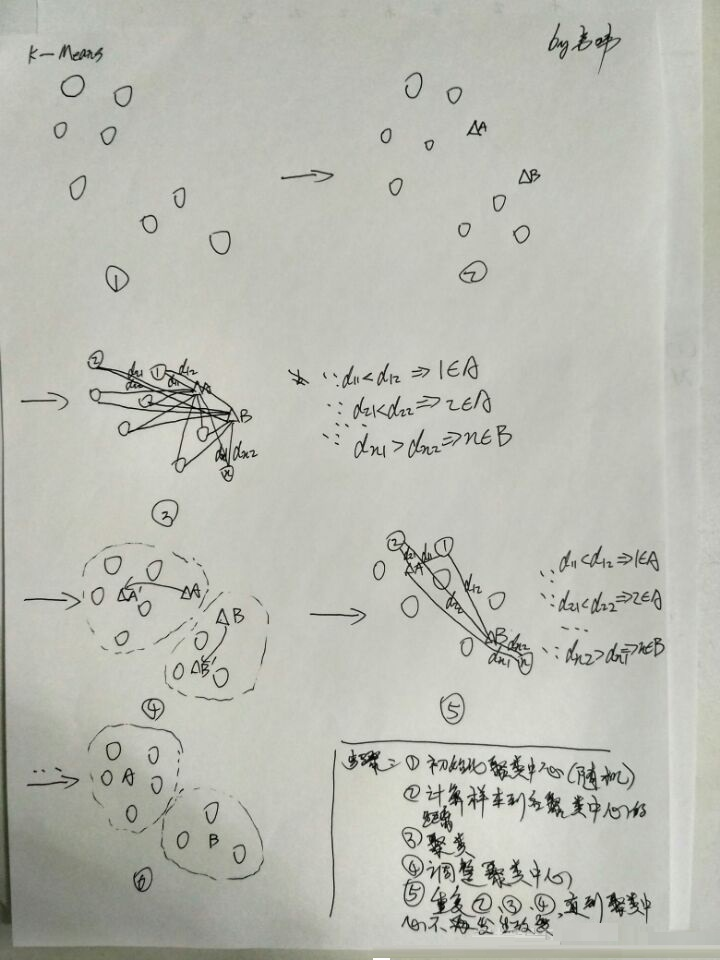from sklearn.cluster import KMeans kms=KMeans(n_clusters=3) y=kms.fit_predict(x)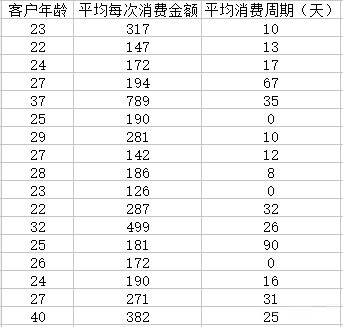#通过聚类分析客户价值 import pandas as pda import numpy as npy import matplotlib.pylab as pyl fname="D:\\我的教学\\Python\\阿里云系列直播\\第4次直播代码\\company.csv" dataf=pda.read_csv(fname,encoding="gbk") x=dataf.as_matrix() from sklearn.cluster import KMeans kms=KMeans(n_clusters=3) y=kms.fit_predict(x) print(y) #年龄-消费金额图，消费时间-消费金额图，年龄-消费时间图 for i in range(0,len(y)): if(y[i]==0): print(str(i)+"大众客户") pyl.subplot(2,3,1) #年龄-消费金额图 pyl.plot(dataf.iloc[i:i+1,0:1].as_matrix(), dataf.iloc [i:i+1,1:2].as_matrix(),"*r") pyl.subplot(2,3,2) #消费时间-消费金额图 pyl.plot(dataf.iloc[i:i+1,2:3].as_matrix(), dataf.iloc [i:i+1,1:2].as_matrix(),"*r") pyl.subplot(2,3,3) #年龄-消费时间图 pyl.plot(dataf.iloc[i:i+1,0:1].as_matrix(), dataf.iloc [i:i+1,2:3].as_matrix(),"*r") elif(y[i]==1): print(str(i)+"超级VIP客户") pyl.subplot(2,3,1) #年龄-消费金额图 pyl.plot(dataf.iloc[i:i+1,0:1].as_matrix(), dataf.iloc[i:i+1,1:2].as_matrix(),"sy") pyl.subplot(2,3,2) #消费时间-消费金额图 pyl.plot(dataf.iloc[i:i+1,2:3].as_matrix(), dataf.iloc[i:i+1,1:2].as_matrix(),"sy") pyl.subplot(2,3,3) #年龄-消费时间图 pyl.plot(dataf.iloc[i:i+1,0:1].as_matrix(), dataf.iloc[i:i+1,2:3].as_matrix(),"sy") elif(y[i]==2): print(str(i)+"VIP客户") pyl.subplot(2,3,1) #年龄-消费金额图 pyl.plot(dataf.iloc[i:i+1,0:1].as_matrix(), dataf.iloc[i:i+1,1:2].as_matrix(),"pb") pyl.subplot(2,3,2) #消费时间-消费金额图 pyl.plot(dataf.iloc[i:i+1,2:3].as_matrix(), dataf.iloc[i:i+1,1:2].as_matrix(),"pb") pyl.subplot(2,3,3) #年龄-消费时间图 pyl.plot(dataf.iloc[i:i+1,0:1].as_matrix(), dataf.iloc[i:i+1,2:3].as_matrix(), "pb") pyl.show()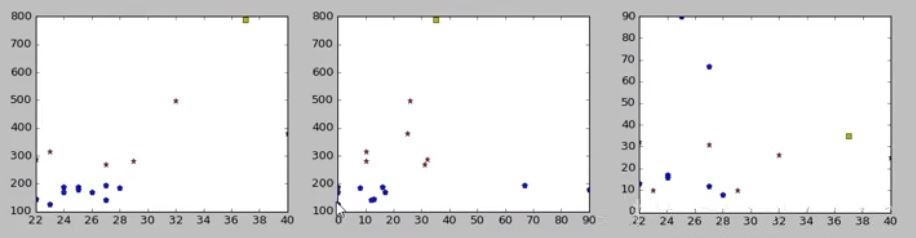Apriori算法是一种基础的关联分析算法，但效率较低，不建议用于商业场景。Apriori算法的原理如下：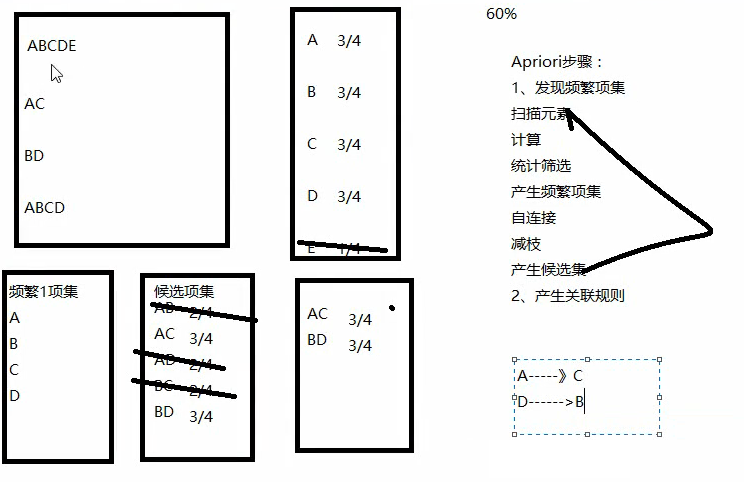Apriori算法为什么效率较低呢？从上述过程可以看到每一次计算候选集的支持度时都需要返回至历史记录中进行扫描，因此处理复杂，耗时严重。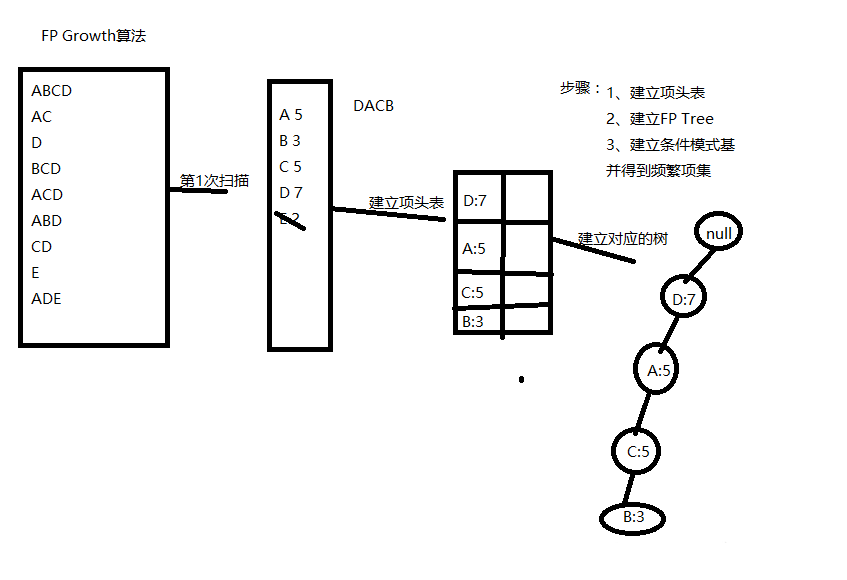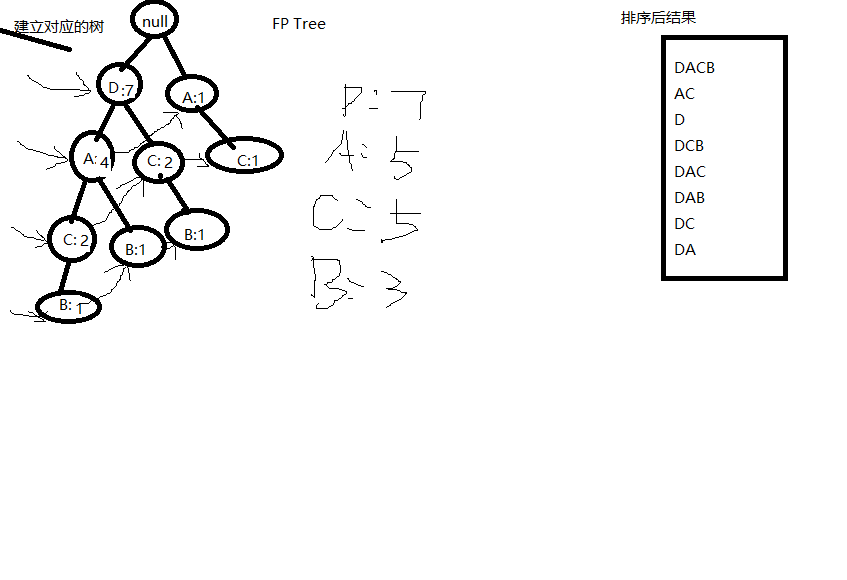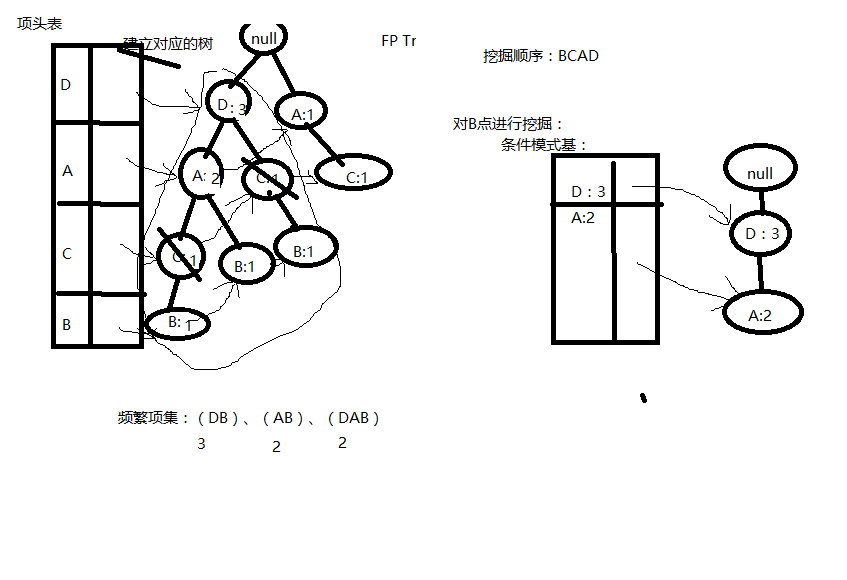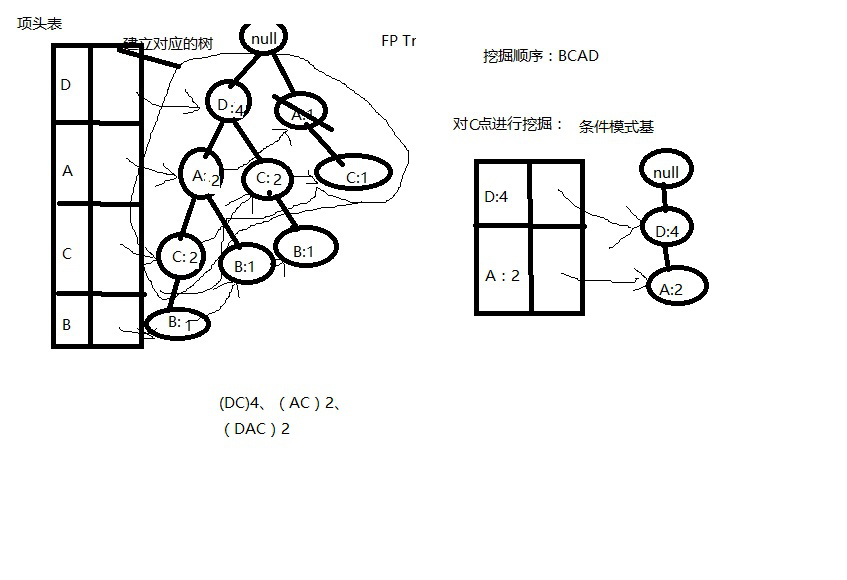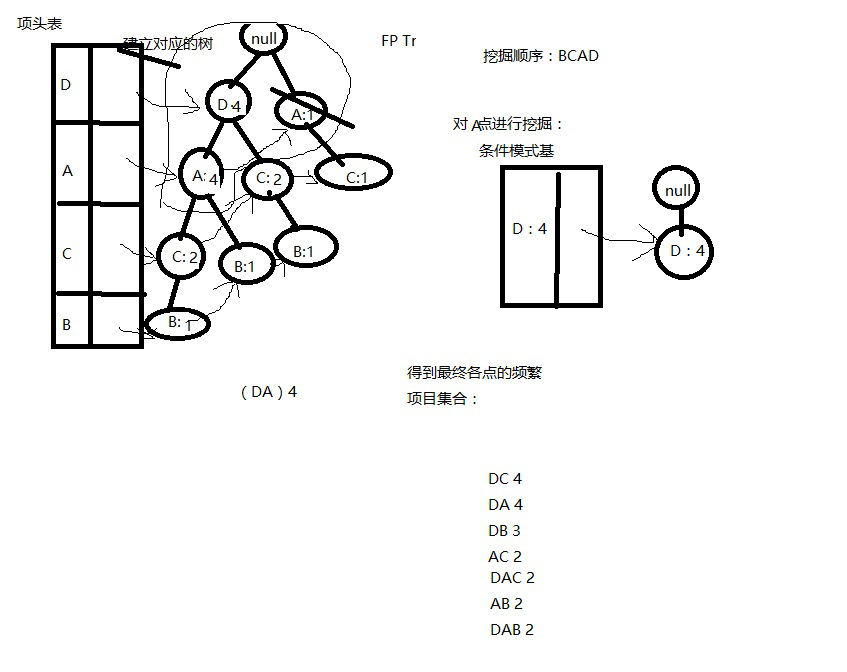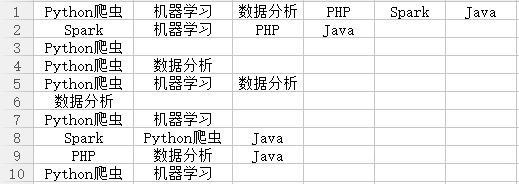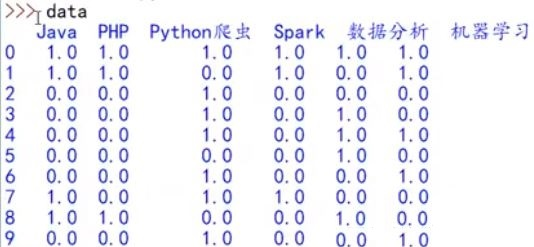from apriori import * import pandas as pda filename="D:/Python35/data/lesson_buy.xls" dframe=pda.read_excel(filename,header=None) change=lambda x:pda.Series(1,index=x[pda.notnull(x)]) mapok=map(change,dframe.as_matrix()) data=pda.DataFrame(list(mapok)).fillna(0) #设置置信度阈值和支持度阈值 surpport=0.2 cfd=0.3 print(find_rule(data,surpport,cfd))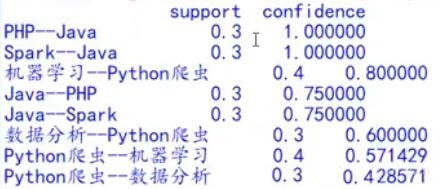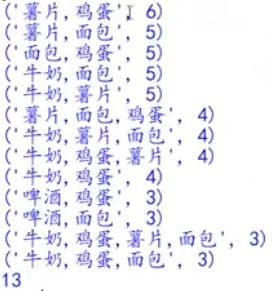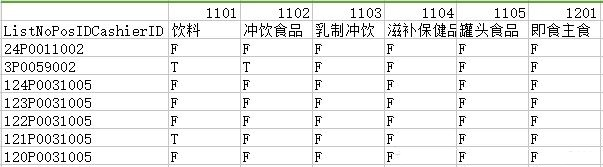1292 次浏览  评价: 好 中 差订阅捐助
 相关文章 手机软件测试用例设计实践 手机客户端UI测试分析 iPhone消息推送机制实现与探讨 Android手机开发（一）
 相关文档 Android_UI官方设计教程 手机开发平台介绍 android拍照及上传功能 Android讲义智能手机开发
 相关课程 Android高级移动应用程序 Android系统开发 Android应用开发 手机软件测试